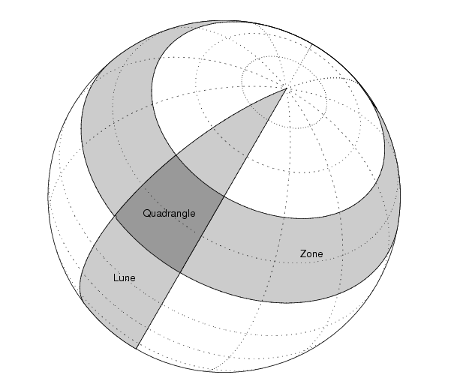## Syntax

```area = areaquad(lat1,lon1,lat2,lon2) area = areaquad(lat1,lon1,lat2,lon2,ellipsoid) area = areaquad(lat1,lon1,lat2,lon2,ellipsoid,units) ```

## Description

`area = areaquad(lat1,lon1,lat2,lon2)` returns the surface area bounded by the parallels `lat1` and `lat2` and the meridians `lon1` and `lon2`. The output `area` is a fraction of the unit sphere's area of 4π, so the result ranges from 0 to 1.

`area = areaquad(lat1,lon1,lat2,lon2,ellipsoid)` allows the specification of the ellipsoid model with `ellipsoid`. `ellipsoid` is a `referenceSphere`, `referenceEllipsoid`, or `oblateSpheroid` object, or a vector of the form ```[semimajor_axis eccentricity]```. When `ellipsoid` is input, the resulting `area` is given in terms of the (squared) units of the ellipsoid. For example, if the ellipsoid `referenceEllipsoid('grs80','kilometers')` is used, the resulting area is in km2.

`area = areaquad(lat1,lon1,lat2,lon2,ellipsoid,units)` where `units` specifies the units of the inputs. The default is `'degrees'`.

## Examples

Find the fraction of the Earth's surface that lies between 30ºN and 45ºN, and also between 25ºW and 60ºE:

```area = areaquad(30,-25,45,60) area = 0.0245```

Assuming a spherical ellipsoid, find the surface area of the Earth in square kilometers.

```earthellipsoid = referenceSphere('earth','km'); area = areaquad(-90,-180,90,180,earthellipsoid) area = 5.1006e+08```

For comparison,

```earthellipsoid.SurfaceArea ans = 5.1006e+08```

collapse all

A latitude-longitude quadrangle is a region bounded by two meridians and two parallels. In spherical geometry, it is the intersection of a lune (a section bounded by two meridians) and a zone (a section bounded by two parallels).## Algorithms

The `areaquad` calculation is exact, being based on simple spherical geometry. For nonspherical ellipsoids, the data is converted to the auxiliary authalic sphere.